# Stationary phase, method of the

(diff) ← Older revision | Latest revision (diff) | Newer revision → (diff)

A method for calculating the asymptotics of integrals of rapidly-oscillating functions: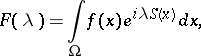(*)

where,,, is a large parameter,is a bounded domain, the function(the phase) is real, the functionis complex, and. If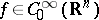, i.e.has compact support, and the phase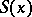does not have stationary points (i.e. points at which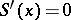) on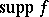,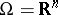, then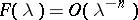, for allas. Therefore, when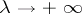, the points of stationary phase and the boundarygive the essential contribution to the asymptotics of the integral (*). The integrals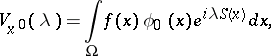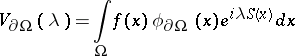are called the contributions from the isolated stationary pointand the boundary, respectively, where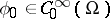,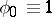near the pointanddoes not contain any other stationary points,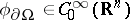andin a certain neighbourhood of the boundary. For,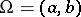:

1)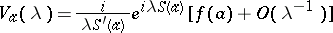, if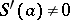;

2)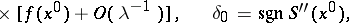if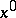is an interior point ofand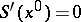,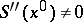.

Detailed research has been carried out in the case where, the phasehas a finite number of stationary points, all of finite multiplicity, and the functionhas zeros of finite multiplicity at these points and at the end-points of an interval. Asymptotic expansions have been obtained. The case where the functionsandhave power singularities has also been studied: for example,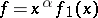,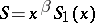, where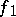,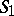are smooth functions when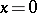,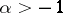,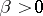.

Let, and let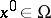be a non-degenerate stationary point (i.e.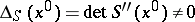). The contribution from the pointis then equal to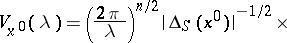whereis the signature of the matrix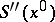. There is also an asymptotic series for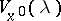(for the formulas of the contributionin the case of a smooth boundary, see ).

Ifis a stationary point of finite multiplicity, then (see )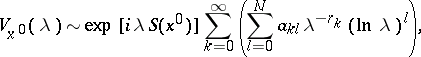where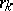are rational numbers,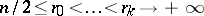. Degenerate stationary points have been studied, cf. , .

Studies have been made on the case where the phase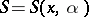depends on a real parameter, and for smallhas two close non-degenerate stationary points. In this case, the asymptotics of the integral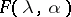can be expressed in terms of Airy functions (see , ). The method of the stationary phase has an operator variant: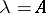, whereis the infinitesimal operator of the strongly-continuous group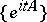of operators bounded on the axis, acting on a Banach space, and,are smooth functions with values in. If the functions are analytic, then the method of the stationary phase is a particular case of the saddle point method.

How to Cite This Entry:
Stationary phase, method of the. Encyclopedia of Mathematics. URL: http://encyclopediaofmath.org/index.php?title=Stationary_phase,_method_of_the&oldid=16013
This article was adapted from an original article by M.V. Fedoryuk (originator), which appeared in Encyclopedia of Mathematics - ISBN 1402006098. See original article